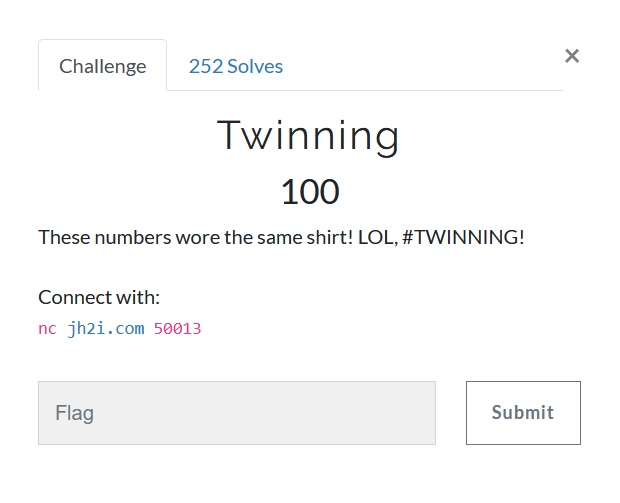# TwinningThe name of the challenge is a subtle hint towards Twin primes, i.e. the RSA primes p and q being twin primes, at a difference of two.

How does that help?
We can now easily factor n since we have another relation, namely

q = p + 2 and
p*q = N
q^2 - q*p = N
( q - 1 )^2 = N + 1
q = sqrt( N + 1 ) + 1
p = sqrt( N + 1 ) - 1


One can easily solve a quadratic equation to find p
Once p and q are found, rest is a piece of cake

Although the time was not a concern, I still wrote a small script

from pwn import remote
import gmpy2
import re

HOST, PORT = "jh2i.com", 50013
REM = remote(HOST, PORT)

data = REM.recvuntil(b'What is the PIN?')
print(data.decode())
e_n = re.search(b'(\d+),(\d+)',data)
e = int(e_n)
n = int(e_n)
PIN = int(re.search(b'is (\d+)',data))

def factor(n,e):
a = gmpy2.iroot(n+1,2)
phi = (a-2)*(a)
return gmpy2.invert(e,phi) # returns d

DECRYPTED_PIN = pow(PIN, factor(n,e), n)
REM.sendline(str(DECRYPTED_PIN).encode())
print(REM.recvline().decode())
print(REM.recvline().decode())
print(REM.recvline().decode())


### flag{thats_the_twinning_pin_to_win}

jekyll.environment != "beta" -%}